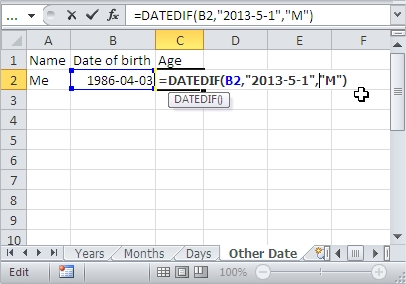## Automatic age calculation

In this lesson you can learn how to calculate date difference between two dates. In an Excel spreadsheet, you can calculate the age based on date of birth.What is interesting is the number of options – values can be expressed in years, months or days. Excel can automatically calculate and display the age on the basis of date of birth.1. In the table put the necessary data, such as name and date of birth.

2. Click on the cell where you want to see a person’s age in years (for example, it is C2). Then in the box type the following formula:

=DATEDIF(B2,TODAY(),”Y”)

B2 is the address of a cell containing a date of birth. Press the Enter key. Age will be calculated as indicated by our cell.In the same way you can show in Excel age in months.

To do this, repeat steps 1 and 2, except that in the formula instead of Y put M – the formula is the following entry:

=DATEDIF(B2,TODAY(),”M”)To calculate Excel the number of days that have passed since a certain date, such as date of birth, also repeat steps 1 and 2, but this time the formula looks like this:

=DATEDIF(B2,TODAY(),”D”)Of course, instead of always served B2 cell address of the date on which Excel is to start counting. Example formula is:

=DATEDIF(B2,”2013-5-1″,”M”)## Template

```Further reading: